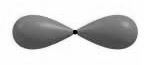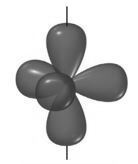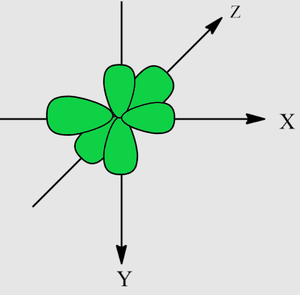# Hybridization – Definition, Types, Rules, Examples

• Last Updated : 07 Dec, 2021

The concept of hybridization is defined as the process of combining two atomic orbitals to create a new type of hybridized orbitals. This intermixing typically results in the formation of hybrid orbitals with completely different energies, shapes, and so on. Hybridization is primarily carried out by atomic orbitals of the same energy level. However, both fully filled and half-filled orbitals can participate in this process if their energies are equal. The concept of hybridization is an extension of valence bond theory that helps us understand the bond formation, bond energies, and bond lengths.

When two atomic orbitals combine to form a hybrid orbital in a molecule, the energy of the orbitals of individual atoms is redistributed to give orbitals of equivalent energy. This is known as hybridization. The atomic orbitals of comparable energies are mixed together during the hybridization process, which mostly involves the merging of two orbitals or two ‘p’ orbitals or the mixing of an ‘s’ orbital with a ‘p’ orbital as well as an ‘s’ orbital with a ‘d’ orbital.

Hybrid orbitals are the new orbitals formed as a result of this process. More importantly, hybrid orbitals can be used to explain atomic bonding properties and molecular geometry. Carbon, for example, forms four single bonds in which the valence-shell s orbital combines with three valence-shell p orbitals. This combination generates four equivalent sp3 mixtures. These will be arranged in a tetrahedral pattern around the carbon, which is bonded to four different atoms.

Features of Hybridization

1. Hybridization occurs between atomic orbitals with equal energies.
2. The number of hybrid orbitals formed equals the number of atomic orbitals that mix.
3. It is not required for all half-filled orbitals to participate in hybridization. Even orbitals that are completely filled but have slightly varying energy can participate.
4. Hybridization occurs only during bond formation, not in a single gaseous atom.
5. If the hybridization of the molecule is known, the shape of the molecule can be predicted.
6. The larger lobe of the hybrid orbital is always positive, while the smaller lobe on the opposite side is always negative.

### Types of Hybridization

Hybridization can be classified as sp3, sp2, sp, sp3d, sp3d2, or sp3d3 based on the types of orbitals involved in mixing.

sp Hybridization

It occurs when one s and one p orbital in an atom’s main shell combine to form two new equivalent orbitals. The newly formed orbitals are known as sp hybridised orbitals. It produces linear molecules with a 180° angle. It entails combining one’s orbital and one ‘p’ orbital of equal energy to produce a new hybrid orbital known as an sp hybridised orbital.

• It’s also known as diagonal hybridization.
• Each sp hybridised orbital contains the same amount of s and p characters.
• All beryllium compounds, such as BeF2, BeH2, and BeCl2, are examples.sp-orbital

sp2 Hybridization

It occurs when one s and two p orbitals of the same atom’s shell combine to form three equivalent orbitals. The newly formed orbitals are known as sp2 hybrid orbitals. It’s also known as trigonal hybridization. It entails combining one’s orbital with two ‘p’ orbitals of equal energy to create a new hybrid orbital known as sp2. A trigonal symmetry mixture of s and p orbitals is kept at 120 degrees. All three hybrid orbitals remain in the same plane and form a 120° angle with one another.

• Each hybrid orbital formed has a 33.33 % and a 66.66 % ‘p’ character.
• The molecules with a triangular planar shape have a central atom that is linked to three other atoms and is sp2 hybridised. Boron compounds are examples.sp2 orbital

sp3 Hybridization

When one ‘s’ orbital and three ‘p’ orbitals from the same shell of an atom combine to form four new equivalent orbitals, the hybridization is known as tetrahedral hybridization or sp3. The newly formed orbitals are known as sp3 hybrid orbitals. These are pointed at the four corners of a regular tetrahedron and form a 109°28′ angle with one another.

• The sp3 hybrid orbitals form a 109.28-degree angle.
• Each hybrid orbital has a 25% s character and a 75% p character.
• Ethane and methane are two examples.sp3 orbital

sp3d Hybridization

The mixing of 1s orbitals, 3p orbitals, and 1d orbitals results in 5 sp3d hybridized orbitals of equal energy. Their geometry is trigonal bipyramidal. The combination of s, p, and d orbitals results in trigonal bipyramidal symmetry. The equatorial orbitals are three hybrid orbitals that are oriented at a 120° angle to each ot

and lie in the horizontal plane.
• The remaining two orbitals, known as axial orbitals, are in the vertical plane at 90 degrees plane of the equatorial orbitals.
• Hybridization in Phosphorus Pentachloride, for example (PCl5).sp3d orbital

#### sp3d2 Hybridization

It has 1s, 3p, and 2d orbitals, which combine to form 6 identical sp3d2 hybrid orbitals. These six orbitals point to the corners of an octahedron. They are inclined at a 90-degree angle to one another.sp3d2 orbital

### Shapes of Hybridization

• Linear: The sp hybridization is caused by the interaction of two-electron groups; the orbital angle is 180°.
• Trigonal planar: Three electron groups are involved, resulting in sp2 hybridization; the orbitals are 120° apart.
• Tetrahedral: Four electron groups are involved, resulting in sp3 hybridization; the orbital angle is 109.5°.
• Trigonal bipyramidal: Five electron groups are involved, resulting in sp3d hybridization; the orbital angles are 90° and 120°.
• Octahedral: Six electron groups are involved, resulting in sp3d2 hybridization; the orbitals are 90° apart.

### Bent’s Rule

A central atom bonded to multiple groups in a molecule will hybridise so that orbitals with more s character are directed towards electropositive groups and orbitals with more p character are directed towards electronegative groups.

Rules for Observing the Type of Hybridisation

To understand the type of hybridisation in a compound or an ion, the following rules must be followed.

1. Calculate the total number of valence electrons.
2. Calculate the number of duplex or octet OR
3. Number of lone pairs of electrons
4. Number of used orbital = Number of duplex or octet + Number of lone pairs of electrons
5. If there is no lone pair of electrons then the geometry of orbitals and molecule is different.

### Sample Questions

Question 1: Among sp, sp2 and sp3, which hybrid orbital is more electronegative?

The percentage of s character in sp, sp2, and sp3 hybridised carbon is 50%, 33.33%, and 25%, respectively. Because of the spherical shape of the s orbital, it is attracted evenly from all directions by the nucleus. As a result, an s-character hybrid orbital will be closer to the nucleus and thus more electronegative. As a result, the sp hybridised carbon is the most electronegative.

Question 2: What are hybrid orbitals?

Hybrid orbitals are formed by combining standard atomic orbitals and resultin

the formation of new atomic orbitals.

Question 3: What are five shapes of hybridization?

Linear, trigonal planar, tetrahedral, trigonal bipyramidal, and octahedral are the five basic shapes of hybridization.

Question 4: Why does the amide molecule look sp3 hybridized but is sp2?